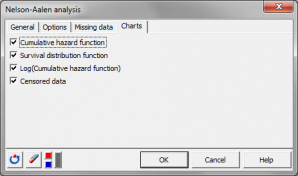# Nelson-Aalen analysis

Nelson-Aalen analysis is a descriptive survival analysis method. Available in Excel using the XLSTAT add-on statistical software.## What is the Nelson-Aalen analysis

The Nelson-Aalen analysis method belongs to the descriptive methods for survival analysis such as life table analysis and Kaplan-Meier analysis. The Nelson-Aalen approach can quickly give you a curve of cumulative hazard and estimate the hazard functions based on irregular time intervals.

Nelson-Aalen analysis is used to analyze how a given population evolves with time. This technique is mostly applied to survival data and product quality data. There are three main reasons why a population of individuals or products may evolve: some individuals die (products fail), some other go out of the surveyed population because they get healed (repaired) or because their trace is lost (individuals move from location, the study is terminated, among other causes) The first type of data is usually called "failure data" or "event data", while the second is called "censored data".

## Censoring of survival data for the Nelson-Aalen analysis

### Censoring types for the Nelson-Aalen analysis

There are several types of censoring of survival data:

• Left censoring: when an event is reported at time t=t(i), we know that the event occurred at t * t(i).
• Right censoring: when an event is reported at time t=t(i), we know that the event occurred at t * t(i), if it ever occurred.
• Interval censoring: when an event is reported at time t=t(i), we know that the event occurred during [t(i-1); t(i)].
• Exact censoring: when an event is reported at time t=t(i), we know that the event occurred exactly at t=t(i).

### Independent censoring for the Nelson-Aalen method

The Nelson-Aalen method requires that the observations are independent. Second, the censoring must be independent – if you consider two random individuals in the study at time t-1, and one of the individuals is censored at time t, while the other survives, then both must have equal chances to survive at time t.

### There are four different types of independent censoring:

• Simple type I: all individuals are censored at the same time or equivalently individuals are followed during a fixed time interval.
• Progressive type I: all individuals are censored at the same date (for example, when the study terminates).
• Type II: the study is continued until n events have been recorded.
• Random: the time when a censoring occurs is independent of the survival time.

## The Nelson-Aalen method and the cumulative hazard function

The Nelson-Aalen analysis allows comparing populations, through their hazards curves. Nelson-Aalen estimator should be preferred to Kaplan-Meier estimator when analyzing cumulative hazard functions. When analyzing cumulative survival functions, Kaplan-Meier estimator should be preferred.

The cumulative hazard function is:

H(T) = ΣTi≤T di/ri

with di being the number of observation falling at time Ti and ri, the number of observation at risk (still in the study) at time Ti.

### Variances for the cumulative hazard function

Several different variance estimators are available:

• Simple
• Plug-in
• Binomial

### Confidence interval for the cumulative hazard function

Confidence intervals can also be obtained:

• Greenwood’s method
• Log-transformed method

The second one will be preferred with small samples.

## XLSTAT results for the Nelson-Aalen analysis

### Nelson-Aalen table

This table displays the various results obtained from the analysis, including:

• Interval start lime: lower bound of the time interval.
• At risk: number of individuals that were at risk.
• Events: number of events recorded.
• Censored: number of censored data recorded.
• Cumulative hazard function: hazard associated with an individual at the considered time.
• Cumulative hazard function error
• Cumulative hazard function confidence interval
• Survival distribution function: probability for an individual to survive until the considered time.

### Charts for the Nelson-Aalen analysis

Depending on the selected options, up to three charts are displayed:

• Cumulative hazard function,
• survival distribution function,
• and Log(Hazard function).

### Test of equality of the survival functions

This table displays the statistics for three different tests:

• the Log-rank test,
• the Wilcoxon test,
• and the Tarone Ware test.

These tests are based on a Chi-square test. The lower the corresponding p-value, the more significant the differences between the groups is.### analyze your data with xlstat

14-day free trial

Included in

Related features